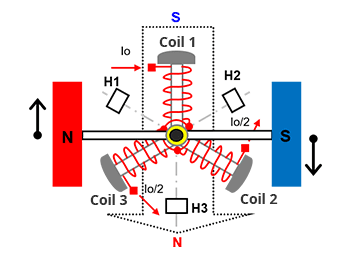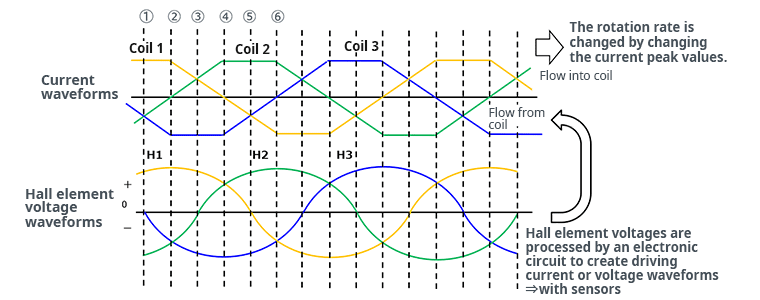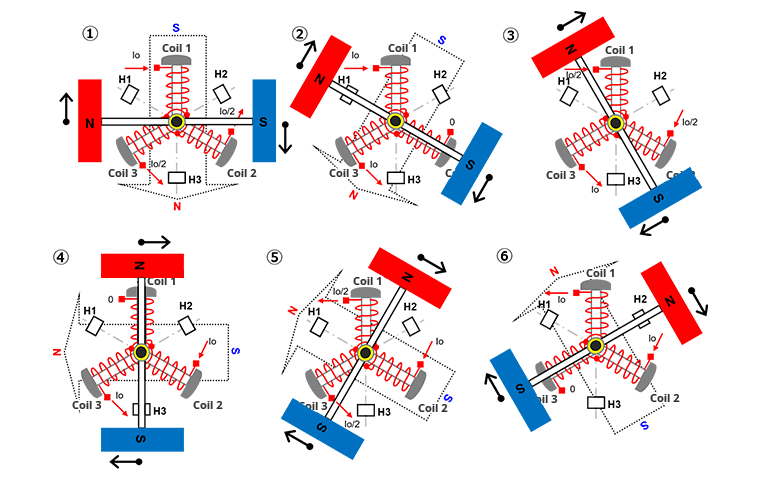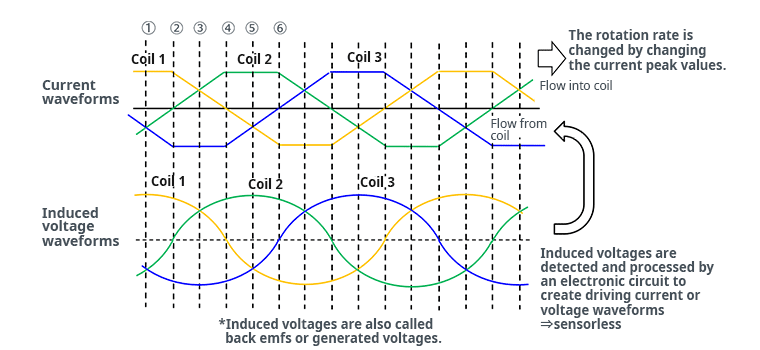# Position Detection in 3-Phase Full-Wave Brushless Motors

2023.06.21

・For position detection in 3-phase full-wave brushless motors, there are methods that use Hall elements, and methods that utilize the voltages induced in the motor coils without relying on Hall elements.

In the previous discussion of “Principles of Rotation of 3-Phase Full-Wave Brushless Motors“, it was explained that a 3-phase full-wave brushless motor rotates as a result of switching of the driving currents supplied to the three coils. Next, we will explain driving methods; but before this, because in actual motor driving the position of the rotating permanent magnet must be detected, we first explain methods of position detection.

There are two main methods of position detection. One is a method using sensors; specifically, the voltages of Hall elements are employed. In the diagrams used to explain the principles of rotation in the previous article, though not explicitly explained, H1, H2, and H3 are Hall elements (sensors). The other method involves detection of the induced voltages for each coil, and does not use sensors, and so is called a sensorless method.

## Position Detection Using Hall Elements (Sensors)

When using Hall elements (sensors) to detect the position of the rotating permanent magnet, the installed positions of the Hall elements are set at the midpoints between coils, that is, at points located at 1/2 the angles between the coils (see the diagram on the right).

If the Hall element between coil 3 and coil 1 is H1, the Hall element between coils 1 and 2 is H2, and the Hall element between coils 2 and 3 is H3, then the signal waveforms of the Hall elements appear as follows (lower waveforms) relative to the current waveforms for clockwise rotation.In this example, when the magnetic field of the N pole is sensed by a Hall element, a positive (+) voltage occurs, and when the S pole magnetic field is sensed a negative (-) voltage is induced; the magnetic field strength changes sinusoidally according to the position of the rotating permanent magnet (the “Hall element voltage waveforms” in the lower part of the waveform diagram). The output current waveforms for each of the phases are taken to be trapezoidal waves (the “current waveforms” in the upper part of the waveform diagram). The numbers ① to ⑥ appearing along the time axis of the waveform diagram correspond to the numbers ① to ⑥ of the diagrams used in the explanation of “Principles of Rotation” in the previous article. The same diagrams are used in the next section on “Position Detection Using Induced Voltages (Sensorless)”, and so can be referenced there.

For motor driving, output current waveforms are synthesized from the Hall element output signal waveforms, which change according to the rotor position. In this synthesis, the H2 voltage waveform is subtracted from the H1 voltage waveform, the H3 voltage waveform is subtracted from the H2 voltage waveform, and the H1 voltage waveform is subtracted from the H3 voltage waveform. Through these operations, sine waves (M1, M2, M3) are obtained that lead H1, H2, H3 in phase by 30°. By generating output currents based on these signals, motor driving current waveforms with the desired phase can be created.

In order to synthesize output current signals to reverse the motor direction, H1 is subtracted from H2, H2 is subtracted from H3, and H3 is subtracted from H1. That is, M1=H2-H1, M2=H3-H2, and M3=H1-H3, and by supplying output currents in accordance with the phases of the synthesized waveforms M1, M2, M3, reverse rotation is obtained.

## Position Detection Using Induced Voltages (Sensorless)

In the method not requiring sensors (Hall elements), induced voltages occurring in the coils are used. In a 3-phase full-wave brushless motor, a permanent magnet rotates relative to the coils and the N pole and S pole change in alternation, so that changes in magnetic flux density occur at the coils, and induced voltages occur in the coils themselves, generating electricity. The magnetic flux density is greatest when the N pole is at a coil end and flux is entering the coil, and when the S pole is at a coil end and flux is leaving the coil. However, when the magnetization of the permanent magnet is sinusoidal, changes in the flux density are greatest at the midpoints between the N pole and the S pole.The above diagrams are the same as those used in the previous article to explain the principles of rotation; the motor states ① to ⑥ correspond to ① to ⑥ on the time axis in the waveform diagram below.The relation between the motor states ① to ⑥ and the induced voltage waveforms are explained below.

• ①： Coil 1 is at a midpoint between the S pole and the N pole, and because the action changes from magnetic flux due to the S pole leaving the outside of the coil to magnetic flux due to the N pole entering the same space, the change in magnetic flux density is greatest. Hence the induced voltage in coil 1 is positive relative to the coil midpoint and is maximum.
• ②： The induced voltage in coil 1 drops, because the change in magnetic flux density is less than before.
• ③： The change in magnetic flux density near coil 1 further decreases, and so the induced voltage also drops further.
• ④： The N pole is at the coil end, and the change in magnetic flux density is zero, so that the induced voltage in coil 1 is zero.
• ⑤： Because the N pole recedes from coil 1, the magnetic flux entering the coil decreases, so that the induced voltage goes negative, but because the change in flux is small, the induced voltage drops a small amount.
• ⑥： The change in the density of magnetic flux entering coil 1 further declines, so that the induced voltage falls still further.

Similarly in coils 2 and 3, at the midpoint when switching from the S pole to the N pole, the maximum positive induced voltage occurs, at the midpoint when switching from the N pole to the S pole the maximum negative induced voltage occurs, and when the N pole or the S pole is at the coil end, the induced voltage is zero.

Moreover, as is shown in the waveform diagram, the induced voltage waveform and the driving current waveform are in phase for each of the coils.

By detecting zero points of the induced voltages and synthesizing output current waveforms, the motor can be driven in rotation using the induced voltages as rotor position detection signals. Hence the motor can be controlled without using Hall elements for position detection.

From the next article, actual driving methods will be explained.

## 【Download Documents】Overview of Brushed DC Motor Structure, Operating Principle, Drive Method, Characteristics, Features, and Applications

Basic overview of brushed motors, including structure, principle of operation, drive method, and features.